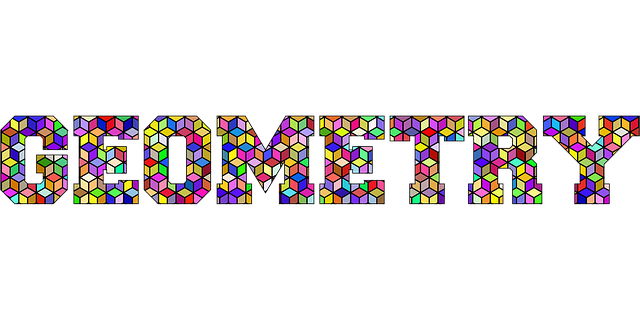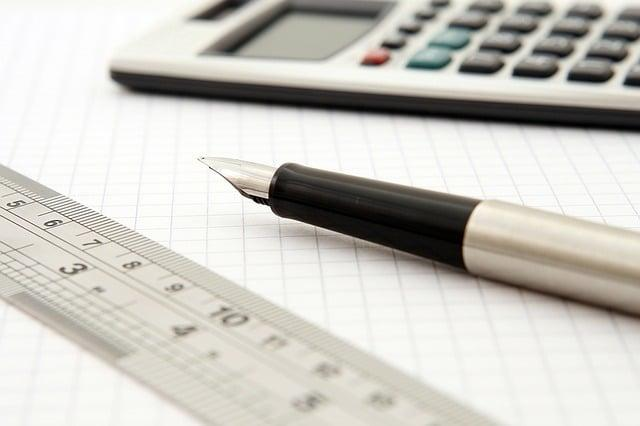Share

# The Foundations of Algebra

By Vanessa, published on 29/08/2019 > > > Discovering the Origins of Algebra

“Algebra is the intellectual instrument which has been created for rendering clear the quantitative aspects of the world.” -Alfred North Whitehead

People’s opinions about algebra are somewhat divided. For example, it’s important to mention that half the population claim that algebra is helpful, and the remaining people couldn’t care less. Individuals who love algebra praise its logic and practicality, while those who couldn’t care less spend time criticising its overall usefulness in everyday life.

What is the correct viewpoint? How should one regard algebra?

While algebra is not everyone’s cup of tea, it is a critical section of mathematics that deserves special attention. By solving algebraic problems, individuals hone their problem solving and critical thinking skills; which are necessary for everyday life.

Those who become familiar with algebra set themselves up for various career opportunities, since it is valued in many distinct fields.

Without further ado, we will briefly consider the foundations of algebra and make it something unusual even for its most avid haters.

## Who Invented Algebra?

Algebra is an essential mathematical topic that has existed for many centuries. Many experienced mathematicians claim that none of the fantastic achievements of modern science would be possible without the mathematisation of science and the development of algebra.

Nevertheless, who is the genius that invented the concepts, rules, and equations associated with algebra?

Algebra stems from the word al-jabr that was initially found in Muhammad ibn Musa al-Khwarizmi’s 9th-century manuscript that when translated means “The Compendious Book on Calculation by Completion and Balancing.”Al-Khwarizmi’s book was a masterpiece that provided practical answers for land distribution, rules on inheritance, and distributing salaries equally.

For example, the term “al-jabr” can be translated as completing or restoring and gives reference to the method of removing a negative from one side and adding the positive to the other.

The father of algebra, Al-Khwarizmi was one of the finest mathematician’s and astronomer’s of his time.

Although Al-Khwarizmi is credited with the earliest theories of algebra, it was not until European mathematicians of the Renaissance that symbolic algebra used today was introduced.

By also developing the concept of the algorithm in mathematics, Al-Khwarizmi is an early pioneer of computer sciences.

## What is Algebra?Learning more about algebra can be frustrating, exhausting, and extremely boring if you do not have a firm foundation of mathematics. (Source: pixabay)

Are you a student who’s continuously bored in math class? Have algebraic problems lost so much of your attention that you question what the point of algebra is?

If you answered yes to both of the previously mentioned questions, you’re not alone; but have no fear, Superprof is here!

A predominant reason why secondary school students strongly dislike algebra is that they don’t exactly know what it is and are unsure of what they are doing. Although completing tutoring sessions with a professional Superprof tutor may still be a viable option and highly recommended option for struggling students, defining algebra may increase understanding and overall appreciation.

The most simple definition of algebra can be understood as the part of mathematics in which letters and symbols are utilised to represent numbers or quantities in formulae and equations.

Doesn’t that sound a bit dull?

To make it sound more interesting, let’s explain it this way: just as distinct sentences describe relationships between different words, in algebra, equations describe relationships between variables.

Algebra can be understood and appreciated as a dialect of mathematical language.

Algebra was initially invented to make life easier for all those practising mathematics; which is contrary to popular belief that it was originated to torment students! Also, it is worthy of mentioning, that throughout the years, pioneers of algebra have worked tirelessly to make algebra more legible.

All in all, algebra is logical and crucial to garnering a further understanding of more complex mathematics.

## What is Linear Algebra?Linear algebra helps all better understand aspects of geometry. (Source: pixabay)

To better comprehend the definition of linear algebra, it is of the utmost importance to analyse the branch of mathematics that concerns linear equations.

What are linear equations?

A linear equation is an equation that may be put in the form of variables and coefficients which are often real numbers. Also, a linear equation provides a straight line when plotted onto a graph.

Therefore, what is linear algebra?

Linear algebra is about linear combinations. Linear algebra is the study of lines and planes, vector spaces, and mappings that are needed for linear transformations.

Therefore, linear algebra is essential to grasp since it is in almost all areas of mathematics. For example, it is necessary for geometry, such as defining objects like lines, planes, and rotations. Also, it is essential to state that linear algebra is used in most sciences and engineering areas.

Many experienced mathematical minds express that without a foundation of linear algebra, it is impossible to develop a deeper understanding of machine learning.

Linear algebra is known by many as the mathematics of data with matrices and vectors being the language of data. Also, linear algebra has made an impact on the field of statistics, and we cannot fail to mention that linear algebra underlies mathematical tools such as Fourier series and computer graphics.

Algebra may seem so complicated at times a tutor should get involved!

## How to Simplify Algebra

Question: what’s more difficult, trying to read Sanskrit or making sense of an algebraic equation? While both are gruelling and extremely complex, those who despise maths would pick anything but algebra.

Nevertheless, what if I told you that there were tricks to make algebra more cognitive and straightforward, would you believe me?

Since algebra has been around for a long time, there have been many improvements and adjustments to make solving equations possible. It is essential to state that to solve an algebraic in the “simplest manner” it needs to be written most compactly and efficiently, without changing the value of the expression.

The most basic rule of simplifying algebraic expressions is knowing that like terms can be added together.

What are like or similiar terms?

Like terms are terms that contain the same powers or same variables; their only difference may be that coefficients change.

To effectively simplify algebraic expressions, the following steps may prove to be quite useful:

• Combining like terms,
• Eliminate the parentheses,
• Minus signs such as subtraction and negatives.

Also, to simplify and correctly execute algebraic expressions, it is essential to remember the Order of Operations in algebra basics; how can this be done? By following the subsequent BEDMAS order:

• B- Brackets,
• E- Exponents,
• D- Division,
• M- Multiplication,
• S- Subtraction.

Also, it is essential to state that those who have not heard of BEDMAS may be familiar with PEMDAS. If you are unsure about certain rules about algebra, consider consulting online resources.

## How to Factorise Algebra

Maths is a complete and layered academic discipline that incorporates so many distinct aspects. For example, factoring is quite essential since it is viewed as the act of finding expressions that multiply together to make a number or equation.

Factoring is an essential skill for those who want to solve algebraic expressions effectively.

By factoring, you are able to solve and eliminate certain possible answers much more quickly than manually.

When factoring numbers and basic algebraic expressions, the following three steps need to be taken into consideration:

1. Correctly understand the definition of factoring when applied to single digits,
2. Remember that variable declarations can also be factored,
3. Apply the distributive property of multiplication.

Also, it is essential to state that there are other specific steps when considering the factorisation of quadratic equations.

## Importance of Learning AlgebraAcquiring the basics of algebra has more pros than cons. (Source: pixabay)

If after reading the previous five subheadings you are still unconvinced about algebra’s overall importance, we strongly urge you to pay attention to the following bulletproof facts:

• More Efficient: many struggling secondary school students do not realise how much more efficient it is to learn algebra than sticking to the elementary aspects of maths. Algebra makes counting variables much more attainable than using other methods and opens up whole new areas of solving life problems such as graphing curves.
• Logical Thinking is Improved: it is essential to state that learning more about algebra is not the only way to teach logic; however, it is a nice-sided benefit since there are two subject areas that reinforce one another.
• Useful Outside of School: while many claim that algebra is never used again outside the academic classroom, it is necessary to mention that this is not exactly true. Many individuals use algebra in specific situations such as determining a health plan, in choosing a cellular phone programme, crafting etc.

Also, algebra is necessary for mastering mathematical subtopics such as statistics and calculus, being more attractive to employers in all sectors, and improving self-confidence, knowing you achieved something great.

In conclusion, being discouraged during algebra classes or harbouring resentment against your maths teacher are no solutions to mastering algebra; hire a Superprof tutor near you today to start learning the right way!

Share(No ratings so far)Loading...